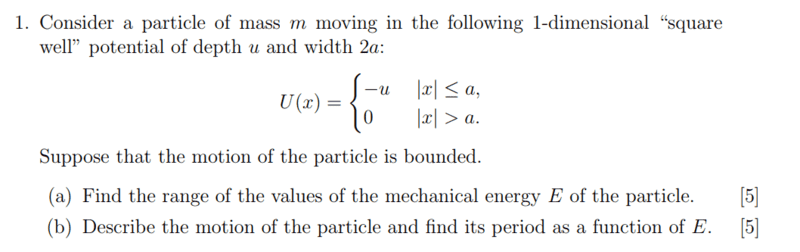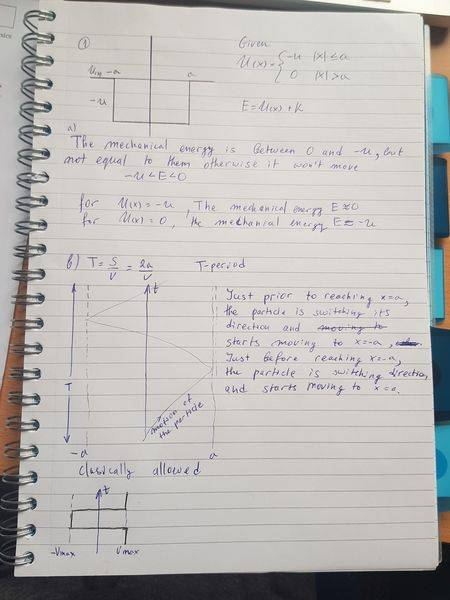# Classical Mechanics Problem: Particle in a Square Potential Well

Jozefina Gramatikova

## Homework Statement

CLASSICAL MECHANICS[/B]

E=U+K[/B]

## The Attempt at a SolutionGuys, can you please help me with part b) ? I am not sure how to find the velocity. Thanks

#### Attachments

Homework Helper
Gold Member
First off, find the range of mechanical energies. What could it possibly be if the motion is bounded? What does "bounded" mean classically?

Jozefina Gramatikova
Is it -u<E<0 ? The motion of the system is bounded if it begins at a point situated between two forbidden regions, the system’s trajectory will never leave the allowed region where it started (otherwise it would have to cross one of the forbidden regions).

Homework Helper
Any relevant equations linking kinetic energy to velocity ?

Jozefina Gramatikova
Could you tell me if this is true?

If U=-u , then E ≈ 0

if U=0, then E ≈ -u

Homework Helper
No, I can tell you it is not true.
U is the potential energy
E is the total energy of the particle, an independent variable in this exercise.
As you almost say (well, guess) in #3, the given information tthat the particle is bounded leads to ##-u \le E < 0##.

With K the kinetic energy and, as you say, E = K + U you can write down the bounds of K and thereby the bounds of v. And derive the period of the motion.

Jozefina Gramatikova
Is it -u<=K<0 ? and then v<0 ?

Jozefina Gramatikova
PLS help

Gordianus
Ah...the curse of negative numbers...
Please, remember K is always a positive number.